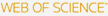# KOPRI Repository

ESTIMATION OF INTRINSIC WAVE PARAMETERS AND MOMENTUM FLUXES OF MESOSPHERIC GRAVITY WAVES OVER KOREA PENINSULA USING ALL-SKY CAMERA AND FABRY-PEROT INTERFEROMETER

Cited 0 time inCited 0 time inTitle
ESTIMATION OF INTRINSIC WAVE PARAMETERS AND MOMENTUM FLUXES OF MESOSPHERIC GRAVITY WAVES OVER KOREA PENINSULA USING ALL-SKY CAMERA AND FABRY-PEROT INTERFEROMETER
Other Titles
전천 카메라와 페브리-페로 간섭계 자료를 이용한 한반도 상공 중간권 중량파의 고유파동계수 및 운동량 플럭스 산출
Authors

Subject
Science & Technology - Other Topics
Keywords
gravity wave; intrinsic wave parameters; momentum flux
Issue Date
2007
Citation
Chung, Jong-Kyun, et al. 2007. "ESTIMATION OF INTRINSIC WAVE PARAMETERS AND MOMENTUM FLUXES OF MESOSPHERIC GRAVITY WAVES OVER KOREA PENINSULA USING ALL-SKY CAMERA AND FABRY-PEROT INTERFEROMETER". The Korean Space Science Society, 24(4): 327-338.
Abstract
We estimate the momentum fluxes of short-period gravity waves which are observed in the OI 557.7 nm nightglow emission with all-sky camera at Mt. Bohyun (36.2$^\circ$ N, 128.9$^\circ$ E) in Korea. The intrinsic phase speed ($C_{int}$), the intrinsic period ($\tau_{int}$), and vertical wavelength ($\lambda_z$) are also deduced from the horizontal wavelength ($\lambda_h$), observed period ($\tau_{ob}$), propagation direction ($\phi_{ob}$), observe phase speed ($\upsilon_{ob}$) of the gravity wave on the all-sky images. The neutral winds to deduce intrinsic wave parameters are measured with Fabry-Perot interferometer on Shigaraki (34.8$^\circ$ N, 13.1$^\circ$ E) in Japan. We selected 5-nights of observations during the period between July 2002 and December 2006 considering of the weather and instrument conditions in two observation sites. The mean values of intrinsic parameter of gravity waves are $(\tau_{int}) = 12.9 \pm 6.1$~m/s, $(\lambda_z) = 12.9 \pm 6.5$, and $(C_{int}) = 40.6 \pm 11.6$ min. The mean value of calculated momentum fluxes for four nights besides of $\lambda_z < 6$ km is $\rm 12.0 \pm 15.2~m^2/s^2$. It is needed the long-term coherent observation to obtain typical values of momentum fluxes of the mesospheric gravity waves using all-sky camera and the neutral wind measurements.
URI
https://repository.kopri.re.kr/handle/201206/6691
DOI
http://dx.doi.org/10.5140/JASS.2007.24.4.327
Files in This Item
##### General Conditions
ROMEO Green
Can archive pre-print and post-print or publisher's version/PDF
ROMEO Blue
Can archive post-print (ie final draft post-refereeing) or publisher's version/PDF
ROMEO Yellow
Can archive pre-print (ie pre-refereeing)
ROMEO White
Archiving not formally supported